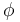# Using symmetries in COMSOL Multiphysics

 Solution Number: 1038 Title: Using symmetries in COMSOL Multiphysics Platform: All Platforms Applies to: All Products Versions: All versions Categories: Physics, Geometry Keywords: symmetry plane axis rotation reflection translation rotoflection rototranslation

## Problem Description

How do I take advantage of the symmetries in my COMSOL model?

## Solution

### Using Symmetries to Reduce Model Size

By using symmetries in a model you can reduce its size by one-half or more, making this an efficient tool for solving large problems. This applies to the cases where the geometries and modeling assumptions include symmetries. The most important types of symmetries are axial symmetry and symmetry and antisymmetry planes or lines.

• Axial Symmetry is common for cylindrical and similar 3D geometries. If the geometry is axisymmetric, there are variations in the radial (r) and vertical (z) direction only and not in the angular () direction. You can then solve a 2D problem in the rz-plane instead of the full 3D model, which can save considerable memory and computation time. Many COMSOL Multiphysics physics interfaces are available in axisymmetric versions and take the axial symmetry into account.
• Symmetry and Antisymmetry Planes or Lines are common in both 2D and 3D models. Symmetry means that a model is identical on either side of a dividing line or plane. For a scalar field, the normal flux is zero across the symmetry line. In structural mechanics, the symmetry conditions are different. Antisymmetry means that the loading of a model is oppositely balanced on either side of a dividing line or plane. For a scalar field, the dependent variable is 0 along the antisymmetry plane or line. Structural mechanics applications have other antisymmetry conditions. Many physics interfaces have symmetry conditions directly available as features.

To take advantage of symmetry planes and symmetry lines, all of the geometry, material properties, and boundary conditions must be symmetric, and any loads or sources must be symmetric or antisymmetric. You can then build a model of the symmetric portion, which can be half, a quarter, or an eighth of the full geometry, and apply the appropriate symmetry (or antisymmetry) boundary conditions.Thermal Contact Resistance Between an Electronic Package and a Heat Sink example with symmetry planes indicated as grey workplanes. Only one sixteenth of the model geometry is used for computing the solution.

#### Examples

For an example of using a symmetry plane in Fluid Dynamics and Heat Transfer, see the model Free Convection.

The above shown model Thermal Contact Resistance Between an Electronic Package and a Heat Sink is available among the Heat Transfer Module examples.

For an example of high-frequency electromagnetic applications with symmetry planes, see the model Microwave Oven from the RF Module.

The model Muffler with Perforates, available in the Acoustics Module, simulates a car muffler with perforated baffles and pipes for a range of frequencies.

#### Possible Pitfalls

In structural mechanics there are some cases when the results are not purely symmetric even though the problem at first may look so.

• Eigenfrequencies in symmetric structures can be both symmetric and antisymmetric. You need to do two studies of half the geometry, one with each set of boundary conditions, in order to capture all the eigenfrequencies. If there are multiple symmetries (like modeling a quarter of a structure) all permutations of boundary conditions must be considered.
• In linearized buckling analysis, the lowest buckling mode of a symmetric structure can in the same way be either symmetric or antisymmetric.
• Axial symmetry can only be used to find axially symmetric eigenmodes in the case of eigenvalue analysis (eigenfrequency or buckling).
• Antisymmetric boundary conditions are generally not compatible with geometrically non-linear analysis of solids since the constraint will remove some strain terms necessary to describe a finite rotation at the
antisymmetry section.